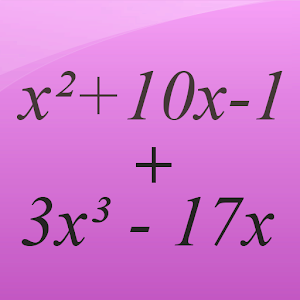## Polynomial Calculator helps you practice Grade HS Math - Lumos Educational App Store3.21
Price -FREE
\$0

#### DESCRIPTION:

This app is a free math calculator which allows you to calculate with polynomials. Yor are able to multiply, divide, add and subtract polynomials. Best mathematical tool for school and college! If you are a student, it will help you to learn algebra! Note: Polynomials appear in settings ranging from basic chemistry and physics to economics and social science; they are used in calculus and numerical analysis to approximate other functions. In advanced mathematics, polynomials are used to construct polynomial rings, a central concept in algebra and algebraic geometry.

#### OVERVIEW:

Polynomial Calculator is a free educational mobile app By GK Apps .It helps students in grades HS practice the following standards A.APR.A.1.

This page not only allows students and teachers download Polynomial Calculator but also find engaging Sample Questions, Videos, Pins, Worksheets, Books related to the following topics.

HS

#### STANDARDS:

A.APR.A.1

Developer: GK Apps

Software Version: 2.9

Category: Education

### RELATED APPSEdSearch WebSearch